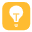240

10

183

156164A+

# mysql分页与Oracle分页

mysql分页采用limit关键字，Oracle采用rownum关键字。

selec * from t limit 1,10;从第一条查询到第十条；（第一页）
selec * from t limit 11,10;从第十条查询到第二十条；（第二页）
selec * from t limit 21,10;从第十条查询到第二十条；（第三页）
ps:记得分页时页数每次加1，不然除了第一次查询是10次，后面的都是9次。

select * from ( select rownum rowno,t.* from test t where rownum <= 10 ) test where test.rowno >= 1;

select * from ( select rownum rowno,t.* from test t where rownum <= 10 ) test where test.rowno >= 1;（第一页）
select * from ( select rownum rowno,t.* from test t where rownum <= 20 ) test where test.rowno >= 11;（第二页）
select * from ( select rownum rowno,t.* from test t where rownum <= 30 ) test where test.rowno >= 21;（第三页）

••友情提示 请保留版权标识

java
30%
javascript
55%
c
10%